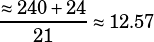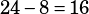Hi Mike. Can you please explain Practise test 1, section 4, question 14? I figured out that the range was the largest change (but only by physically calculating the mean and median etc.)

Is there a better way to do this?

Also, I’d just like to say THANK YOU THANK YOU THANK YOU for this book it really is amazing!!

Well, you have to consider every answer choice, but there are a few mental shortcuts that I think are helpful.

The main thing to recognize right away is that the data is in order, so calculating the median is very easy. The middle value is 12, and if you remove that outlier 24, the median will STILL be 12. (It’s useful to remember that, in general, outliers have little to no effect on the median—that’s why you usually hear about median household income instead of mean household income, for example.) So I can cross of median without calculating it, and then I can cross off choice D as well.

The data being neatly sorted also helps one to think about the mean. Notice how nicely clustered the data is: how the 13s, 14s and 15s pretty nicely balance out the 11s, 10s, and 9s. That tells you that the mean might not be exactly the same as the median, but it should still be very close to 12. So without adding up all the values, I know the average of 20 of the values should be about 12, which means their sum should be about 240 (average times # of numbers equals sum). Adding the erroneous 24 value in there won’t change the average much:. (Note that while this estimate is not completely accurate, it doesn’t matter because you’re just looking for the biggest change, not the exact change.)

The range, on the other hand, will change a lot! The range with the 24 measure in there is. Take that 24 out and the range becomes. That’s by far the biggest change.

PS: Glad you like the Math Guide! 🙂